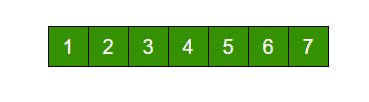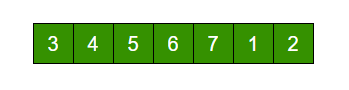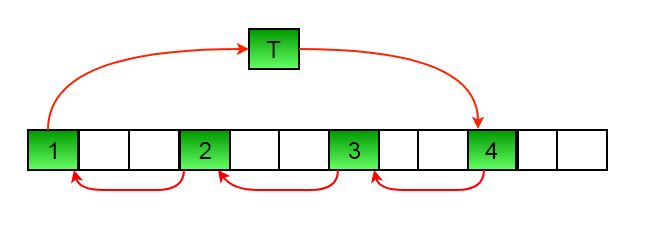# Python Program for array rotation

• Difficulty Level : Medium
• Last Updated : 19 Feb, 2022

Write a function rotate(ar[], d, n) that rotates arr[] of size n by d elements.Rotation of the above array by 2 will make arrayMETHOD 1 (Using temp array):

```Input arr[] = [1, 2, 3, 4, 5, 6, 7], d = 2, n =7
1) Store d elements in a temp array
temp[] = [1, 2]
2) Shift rest of the arr[]
arr[] = [3, 4, 5, 6, 7, 6, 7]
3) Store back the d elements
arr[] = [3, 4, 5, 6, 7, 1, 2]```

## Python3

 `# function to rotate array by d elements using temp array` `def` `rotateArray(arr, n, d):` `    ``temp ``=` `[]` `    ``i ``=` `0` `    ``while` `(i < d):` `        ``temp.append(arr[i])` `        ``i ``=` `i ``+` `1` `    ``i ``=` `0` `    ``while` `(d < n):` `        ``arr[i] ``=` `arr[d]` `        ``i ``=` `i ``+` `1` `        ``d ``=` `d ``+` `1` `    ``arr[:] ``=` `arr[: i] ``+` `temp` `    ``return` `arr`     `# Driver function to test above function` `arr ``=` `[``1``, ``2``, ``3``, ``4``, ``5``, ``6``, ``7``]` `print``(``"Array after left rotation is: "``, end``=``' '``)` `print``(rotateArray(arr, ``len``(arr), ``2``))`   `# this code is contributed by Anabhra Tyagi`

Output

`Array after left rotation is:  [3, 4, 5, 6, 7, 1, 2]`

Time complexity: O(n)
Auxiliary Space: O(d)

METHOD 2 (Rotate one by one) :

```leftRotate(arr[], d, n)
start
For i = 0 to i < d
Left rotate all elements of arr[] by one
end```

To rotate by one, store arr in a temporary variable temp, move arr to arr, arr to arr …and finally temp to arr[n-1]
Let us take the same example arr[] = [1, 2, 3, 4, 5, 6, 7], d = 2
Rotate arr[] by one 2 times
We get [2, 3, 4, 5, 6, 7, 1] after first rotation and [ 3, 4, 5, 6, 7, 1, 2] after second rotation.

## Python3

 `#Function to left rotate arr[] of size n by d*/` `def` `leftRotate(arr, d, n):` `    ``for` `i ``in` `range``(d):` `        ``leftRotatebyOne(arr, n)`   `#Function to left Rotate arr[] of size n by 1*/ ` `def` `leftRotatebyOne(arr, n):` `    ``temp ``=` `arr[``0``]` `    ``for` `i ``in` `range``(n``-``1``):` `        ``arr[i] ``=` `arr[i``+``1``]` `    ``arr[n``-``1``] ``=` `temp` `        `    `# utility function to print an array */` `def` `printArray(arr,size):` `    ``for` `i ``in` `range``(size):` `        ``print` `(``"%d"``%` `arr[i],end``=``" "``)`   ` `  `# Driver program to test above functions */` `arr ``=` `[``1``, ``2``, ``3``, ``4``, ``5``, ``6``, ``7``]` `leftRotate(arr, ``2``, ``7``)` `printArray(arr, ``7``)`   `# This code is contributed by Shreyanshi Arun`

Output

`3 4 5 6 7 1 2 `

Time complexity : O(n * d)
Auxiliary Space : O(1)

METHOD 3 (A Juggling Algorithm)
This is an extension of method 2. Instead of moving one by one, divide the array in different sets
where number of sets is equal to GCD of n and d and move the elements within sets.
If GCD is 1 as is for the above example array (n = 7 and d =2), then elements will be moved within one set only, we just start with temp = arr and keep moving arr[I+d] to arr[I] and finally store temp at the right place.
Here is an example for n =12 and d = 3. GCD is 3 and

```Let arr[] be {1, 2, 3, 4, 5, 6, 7, 8, 9, 10, 11, 12}

a)    Elements are first moved in first set – (See below diagram for this movement``````          arr[] after this step --> {4 2 3 7 5 6 10 8 9 1 11 12}

b)    Then in second set.
arr[] after this step --> {4 5 3 7 8 6 10 11 9 1 2 12}

c)    Finally in third set.
arr[] after this step --> {4 5 6 7 8 9 10 11 12 1 2 3}```

## Python3

 `#Function to left rotate arr[] of size n by d` `def` `leftRotate(arr, d, n):` `    ``for` `i ``in` `range``(gcd(d,n)):` `        `  `        ``# move i-th values of blocks ` `        ``temp ``=` `arr[i]` `        ``j ``=` `i` `        ``while` `1``:` `            ``k ``=` `j ``+` `d` `            ``if` `k >``=` `n:` `                ``k ``=` `k ``-` `n` `            ``if` `k ``=``=` `i:` `                ``break` `            ``arr[j] ``=` `arr[k]` `            ``j ``=` `k` `        ``arr[j] ``=` `temp`   `#UTILITY FUNCTIONS` `#function to print an array ` `def` `printArray(arr, size):` `    ``for` `i ``in` `range``(size):` `        ``print` `(``"%d"` `%` `arr[i], end``=``" "``)` ` `  `#Function to get gcd of a and b` `def` `gcd(a, b):` `    ``if` `b ``=``=` `0``:` `        ``return` `a;` `    ``else``:` `        ``return` `gcd(b, a``%``b)` ` `  `# Driver program to test above functions ` `arr ``=` `[``1``, ``2``, ``3``, ``4``, ``5``, ``6``, ``7``]` `leftRotate(arr, ``2``, ``7``)` `printArray(arr, ``7``)`   `# This code is contributed by Shreyanshi Arun`

Output

`3 4 5 6 7 1 2 `

Time complexity : O(n)
Auxiliary Space : O(1)

Another Approach : Using List slicing

## Python3

 `# Python program using the List ` `# slicing approach to rotate the array` `def` `rotateList(arr,d,n):` `  ``arr[:]``=``arr[d:n]``+``arr[``0``:d]` `  ``return` `arr` `# Driver function to test above function ` `arr ``=` `[``1``, ``2``, ``3``, ``4``, ``5``, ``6``] ` `print``(arr) ` `print``(``"Rotated list is"``) ` `print``(rotateList(arr,``2``,``len``(arr)))  `   `# this code is contributed by virusbuddah`

Output

```[1, 2, 3, 4, 5, 6]
Rotated list is
[3, 4, 5, 6, 1, 2]```

If array needs to be rotated by more than its length then mod should be done.

For example: rotate arr[] of size n by d where d is greater than n. In this case d%n should be calculated and rotate by the result after mod.
Please refer complete article on Program for array rotation for more details!

My Personal Notes arrow_drop_up
Recommended Articles
Page :